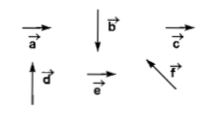NEET Questions Solved

NEET - 2010

Six vector  have the directions indicated in the figure.Which of the following statements may be true?(a) $\stackrel{\to }{b}+\stackrel{\to }{c}=\stackrel{\to }{-f}$                        (b) $\stackrel{\to }{d}+\stackrel{\to }{c}=\stackrel{\to }{f}$

(c) $\stackrel{\to }{d}+\stackrel{\to }{e}=\stackrel{\to }{f}$                        (d) $\stackrel{\to }{b}+\stackrel{\to }{e}=\stackrel{\to }{f}$

If two non-zero vectors are represented by the two adjust sides of a parallelogram, then the resultant is given by the diagonal of the parallelogram passing through the point of intersection of the two vectors

Difficulty Level:

• 38%
• 25%
• 23%
• 15%